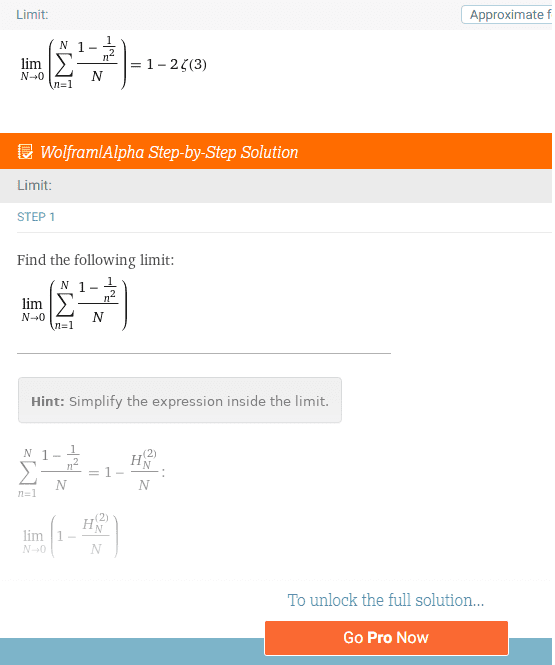# Summation from 1 to .... 0?

• I
• AVentura

#### AVentura

TL;DR Summary
The physical meaning (if any) of this type of summation.
Hello, I was finding the average value of the expression ##(1-1/n^2)## for values from 1 to infinity by evaluating the limit as N→∞ for:

## \displaystyle\sum_{n=1}^{N} (1-1/n^{2})/N ##

and got what I expected, ##1##

What I didn't expect was to find that the general solution ##1-H_N^{(2)}/N## provided real values for ##N < 1##

Assuming that ##(1-1/n^2)## represents something physical, as in a statistical mechanics problem, what does it mean to summate from 1 upwards to something less than 1?

Thank you

Last edited:

That does not make any sense, I suggest you check your ## \LaTeX ##. What is ## H_N ##? It will be clearer if you write fractions using the \frac{}{} notatation: ## 1 - \frac{1}{n^2} ##.
what does it mean to summate from 1 upwards to something less than 1?
It doesn't mean anything: clearly if ## N < 1 ## you cannot reach ## n = N ## by starting at ## n = 1 ## and moving upwards.

•Klystron
Thank you,

##H_n^{(r)}## is the generalized harmonic number

$$\lim_{N→0} [1-\frac{H_N^{(2)}}{N}]=1-2\zeta(3)≈-1.40411$$
where ##\zeta## is the Riemann zeta function.

So to recap
$$\lim_{N→0} \displaystyle\sum_{n=1}^{N} \frac{(1-\frac{1}{n^{2}})}{N}≈-1.40411$$

or maybe it is clearer this way:

$$\lim_{N→0} \frac{\displaystyle\sum_{n=1}^{N} (1-\frac{1}{n^{2}})}{N}≈-1.40411$$

How could ##N## arrive at zero? Left turn at Albuquerque?

It doesn't mean anything: clearly if ## N < 1 ## you cannot reach ## n = N ## by starting at ## n = 1 ## and moving upwards.

Physical meanings aside, it seems mathematically speaking even if ##N<1## one can reach ## n = N ## by starting at ## n = 1 ## and moving upwards.

Last edited:
or maybe it is clearer this way:

$$\lim_{N→0} \frac{\displaystyle\sum_{n=1}^{N} (1-\frac{1}{n^{2}})}{N}≈-1.40411$$
I don't see how the summation here makes any sense. The index, n, starts off at 1, and increases in integer steps to N, which in a summation is assumed to be greater than or equal to the starting value of the index.

•pbuk
Yes, I agree. Perhaps someone could have a look at wolfram alpha's step by step proof for this answer. I must admit it is beyond me.

##H_n^{(r)}## is the generalized harmonic number

$$\lim_{N→0} [1-\frac{H_N^{(2)}}{N}]=1-2\zeta(3)≈-1.40411$$
where ##\zeta## is the Riemann zeta function.
Check that and provide a reference please.

Yes, I agree. Perhaps someone could have a look at wolfram alpha's step by step proof for this answer. I must admit it is beyond me.
Are you referring to equation 23 on the page on harmonic numbers? Where is your sum from ## 1..\infty ##?

I did see that, and I assume it's used somehow in the result I posted. I used wolfram's solver directly. I don't have "pro" so I can't see the steps.

I guess wolframalpha is just making a mistake. Perhaps it solves an intermediate step and then applies a limit that violates a previous step?

but $$\lim_{N→0} [1-\frac{H_N^{(2)}}{N}]=1-2\zeta(3)≈-1.40411$$
is very believable. ## H_N^{(2)} ## goes to zero as ##N## does. Just at a different rate.

Last edited:
No, this is nonsense. Given the two possibilities
1. Wolfram Alpha is making a mistake
2. You are making a mistake in your input to Wolfram Alpha (or your interpretation of the result)
I know which I find more believable. Why don't you post the link to the result?

https://www.wolframalpha.com/input/?i=lim+N->0+{∑(1-1/n^2)/N+,+n=1,N}Are we seeing the error right after the first step?
btw, WA gives real solutions for all ##N##, except the negative integers, which seem to all be negative infinity.

looks like there are less complicated ways to make WA do something like this:
https://www.wolframalpha.com/input/?i=∑n+,+n=1,N+,+N=0.5

Last edited:
•pbuk
The confusion is now clear.

For positive integer values of ## n ##, we define the generalised harmonic number $$H_n^{(r)} = \sum_{k=1}^n \frac1{k^r} \tag 1$$
(note that it helps to avoid confusion by avoiding using ## n ## for the index of summation and ## N ## for the limit).

We can also find a continuous function ## H(x, r) ## which interpolates the values of ## H_n^{(r)} ## at positive integer ## n ##; this function is described in the references to the linked Wolfram Alpha article. It can be shown that $$\lim_{x \to 0} \frac{H(x, 2)}{x} = 2\zeta(3) \tag 2$$ and this is indeed an interesting result.

However what you can't do is put these two together: equation (1) tells us about what happens at positive integer values, equation (2) tells us about what happens near 0.

Last edited:
•Mark44
Are we seeing the error right after the first step?
btw, WA gives real solutions for all ##N##, except the negative integers, which seem to all be negative infinity.

looks like there are less complicated ways to make WA do something like this:
https://www.wolframalpha.com/input/?i=∑n+,+n=1,N+,+N=0.5
Garbage In, Garbage Out.

Thank you for your help clearing this up.

Have you seen in recent pop media the claim that ##1+2+3 ... \infty = -\frac {1} {12}## ?
I'm guessing it is a similar mistake. People are using the definition for the zeta function > 1 for the point s = -1.

Yes, there are a number of threads in this forum on that topic.

Analytic number theory is a fascinating branch of mathemetics about which I am afraid I know very little, but I know an abuse of notation when I see one!

•AVentura